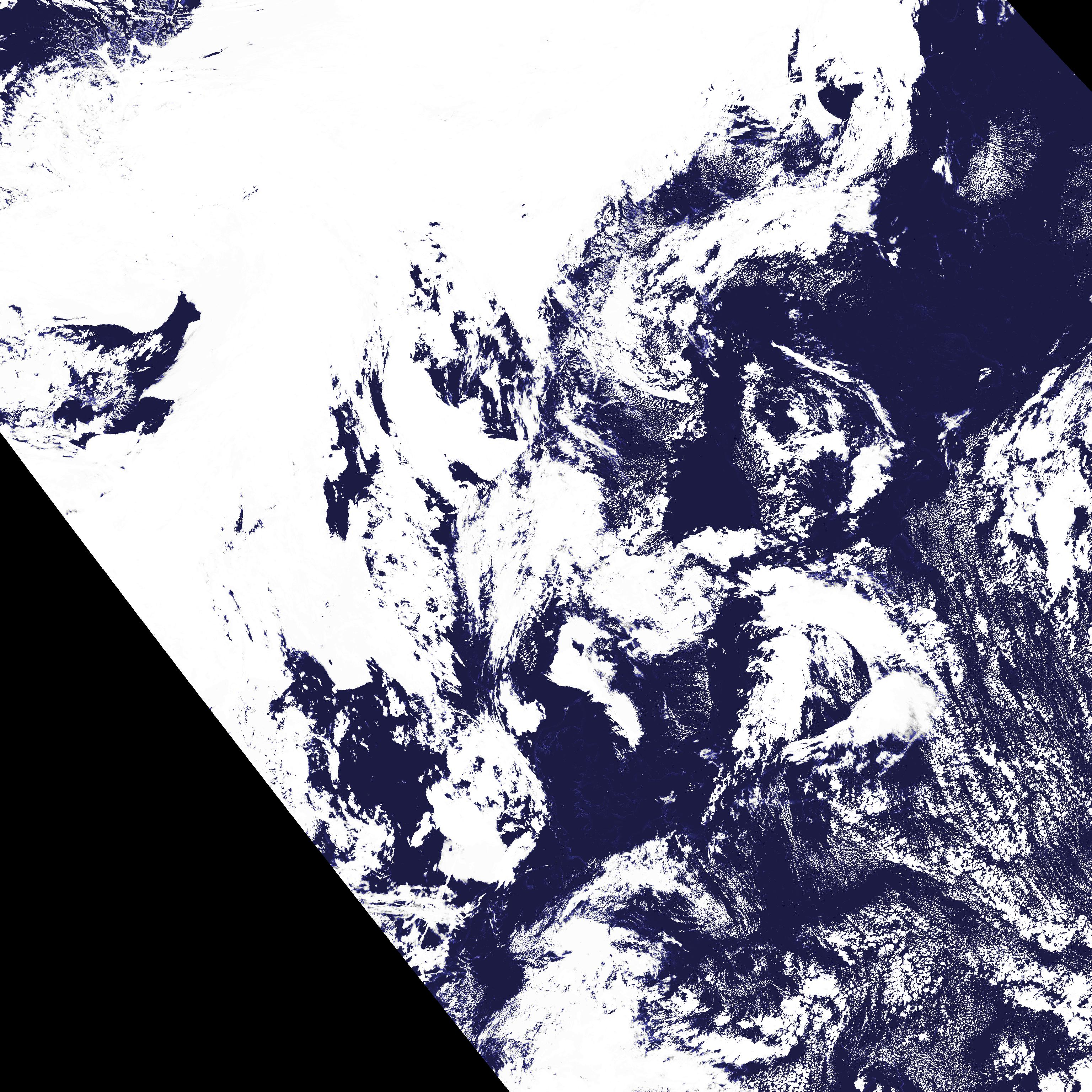# Cloud Probability (CMa-prob)

Contents:## Goal of the Cloud Probability product

This product provides cloud probabilities. I.e., each value on pixel level gives the likelihood in percent, that this pixel is cloudy.

The product can be used as a binary cloud mask by applying a threshold on the cloud probability. Depending on where you choose to set this limit/threshold, the cloud mask can range from clear-conservative to cloud-conservative or anything in between. It can also be seen as a binary cloud mask, with a built-in error estimate.

## Cloud Probability algorithm summary

The cma-prob algorithm uses the naïve Bayes approach to estimate the cloud probability of individual pixels. The dynamic threshold tables from the classic (binary) approach are used to simplify the problem. The algorithm takes satellite imagery input from the sensors avhrr, viirs or modis, on board the following satellite platforms: NOAA, Metop, SNPP/JPSS, and EOS-Terra/Aqua. Besides the satellite data the classical thresholding algorithm for the binary mask, takes the following as input:

• nwp short range forecast data
• 1 km gis (digital elevation model and landuse) data
• Land emissivity maps

## Cloud Probability output

The Cloud Probability output consists of:

• a cloud probability dataset
• three quality/conditions flags

### Main output

The main output is the cloud probability dataset:

• Cloud Probability in percent.